Determine the numbers b, c that the numbers x1 = -1 and x2 = 3 were roots of quadratic equation:

$-3{x}^{2}+bx+c=0$

b =  6
c =  9

### Step-by-step explanation: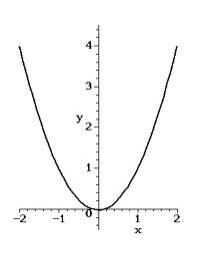Did you find an error or inaccuracy? Feel free to write us. Thank you!Tips to related online calculators

## Related math problems and questions:

• Equation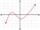Eequation f(x) = 0 has roots x1 = 64, x2 = 100, x3 = 25, x4 = 49. How many roots have equation f(x2) = 0 ?
• Roots count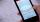Substitute the numbers/0,1,2,3/into the equation as x: (x - 1) (x - 3) (x + 1) = 0 Which of them is its solution? Is there another number that solves this equation?
• CoordinatesDetermine the coordinates of the vertices and the content of the parallelogram, the two sides of which lie on the lines 8x + 3y + 1 = 0, 2x + y-1 = 0 and the diagonal on the line 3x + 2y + 3 = 0
• Roots and coefficient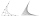In the equation 2x ^ 2 + bx-9 = 0 is one root x1 = -3/2. Determine the second root and the coefficient b.
• EquationEquation -2x2+bx -82 =0 has one root x1 = -8. Determine the coefficient b and the second root x2.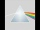Please determine the solvability conditions of the equation, solve the equation and perform the test: x divided by x squared minus 2x plus1 the whole minus x + 3 divided by x squared minus 1 this is equal to 0: x/(x2-2x+1) - (x+3)/( x2-1) = 0
• Find the 20Find the product and the sum of the roots of x2 + 3x - 9 = 0Find the roots of the quadratic equation: 3x2-4x + (-4) = 0.
• What is 10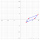What is this The area of a parallelogram that has vertices with the coordinates (0, 0), (4, 0),(5, 3), and (1, 3)?
• Sphere from tree pointsEquation of sphere with three point (a,0,0), (0, a,0), (0,0, a) and center lies on plane x+y+z=a
• Intersections 3Find the intersections of the circles x2 + y2 + 6 x - 10 y + 9 = 0 and x2 + y2 + 18 x + 4 y + 21 = 0
• Sorting ASCWhich group of decimals is in order from least to greatest? A) 1.04, 0.39, 0.8, 2.1, 0.09 B) 2.1, 1.04, 0.39, 0.8, 0.09 C) 0.09, 0.39, 0.8, 1.04, 2.1 D) 0.09, 0.39, 0.8, 2.1, 1.04
• Vector equationLet’s v = (1, 2, 1), u = (0, -1, 3) and w = (1, 0, 7) . Solve the vector equation c1 v + c2 u + c3 w = 0 for variables c1 c2, c3 and decide weather v, u and w are linear dependent or independent
• Probability of intersectionThree students have a probability of 0.7,0.5 and 0.4 to graduated from university respectively. What is the probability that at least one of them will be graduated?
• Solve 2Solve integer equation: a +b+c =30 a, b, c = can be odd natural number from this set (1,3,5,7,9,11,13,15)
• Subtract 4Subtract the following decimals. a. 0.98 - 0.053 b. 0.67 - 0.4 c. 0.3 - 0.002 d. 3.2 - .789 e. 6.53 - 4.298 f. 6 - 4.32 g. 7 - 3.574 h. 4.83 - 1.8 i. 3.7 - 1.8 j. 16.17 - 11.632
• Phone numbersHow many 7-digit telephone numbers can be compiled from the digits 0,1,2,..,8,9 that no digit is repeated?